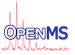OpenMS
BSpline2d Class Reference

b spline interpolation More...

#include <OpenMS/MATH/MISC/BSpline2d.h>

Collaboration diagram for BSpline2d:
[legend]

## Public Types

enum  BoundaryCondition { BC_ZERO_ENDPOINTS = 0 , BC_ZERO_FIRST = 1 , BC_ZERO_SECOND = 2 }

## Public Member Functions

BSpline2d (const std::vector< double > &x, const std::vector< double > &y, double wavelength=0, BoundaryCondition boundary_condition=BC_ZERO_SECOND, Size num_nodes=0)

virtual ~BSpline2d ()

bool solve (const std::vector< double > &y)

double eval (const double x) const

double derivative (const double x) const

bool ok () const

## Static Public Member Functions

static void debug (bool enable)

## Private Attributes

eol_bspline::BSpline< double > * spline_

## Detailed Description

b spline interpolation

## ◆ BoundaryCondition

 enum BoundaryCondition
Enumerator
BC_ZERO_ENDPOINTS

Set the endpoints of the spline to zero.

BC_ZERO_FIRST

Set the first derivative of the spline to zero at the endpoints.

BC_ZERO_SECOND

Set the second derivative to zero.

## ◆ BSpline2d()

 BSpline2d ( const std::vector< double > & x, const std::vector< double > & y, double wavelength = 0, BoundaryCondition boundary_condition = BC_ZERO_SECOND, Size num_nodes = 0 )

Create a single spline with the parameters required to set up the domain and subsequently smooth the given set of y values. The y values must correspond to each of the x values. If either the domain setup fails or the spline cannot be solved, the state will be set to "not OK".

ok().
Parameters
 x The array of x values in the domain. y The array of y values corresponding to each of the x values in the domain. wavelength The cutoff wavelength, in the same units as the x values. A wavelength of zero disables the derivative constraint. boundary_condition The boundary condition type. If omitted it defaults to BC_ZERO_SECOND. num_nodes The number of nodes to use for the cubic b-spline. If less than 2, a "reasonable" number will be calculated automatically, taking into account the given cutoff wavelength.
Precondition
x and y must be of the same dimensions.

## ◆ ~BSpline2d()

 virtual ~BSpline2d ( )
virtual

Destructor

## ◆ debug()

 static void debug ( bool enable )
static

Enable or disable debug messages from the B-spline library.

## ◆ derivative()

 double derivative ( const double x ) const

Return the first derivative of the spline curve at the given x. Returns zero if the current state is not ok().

## ◆ eval()

 double eval ( const double x ) const

Return the evaluation of the smoothed curve at a particular x value. If current state is not ok(), returns zero.

## ◆ ok()

 bool ok ( ) const

Return whether the spline fit was successful.

## ◆ solve()

 bool solve ( const std::vector< double > & y )

Solve the spline curve for a new set of y values. Returns false if the solution fails.

Parameters
 y The array of y values corresponding to each of the x values in the domain.

## ◆ spline_

 eol_bspline::BSpline* spline_
private